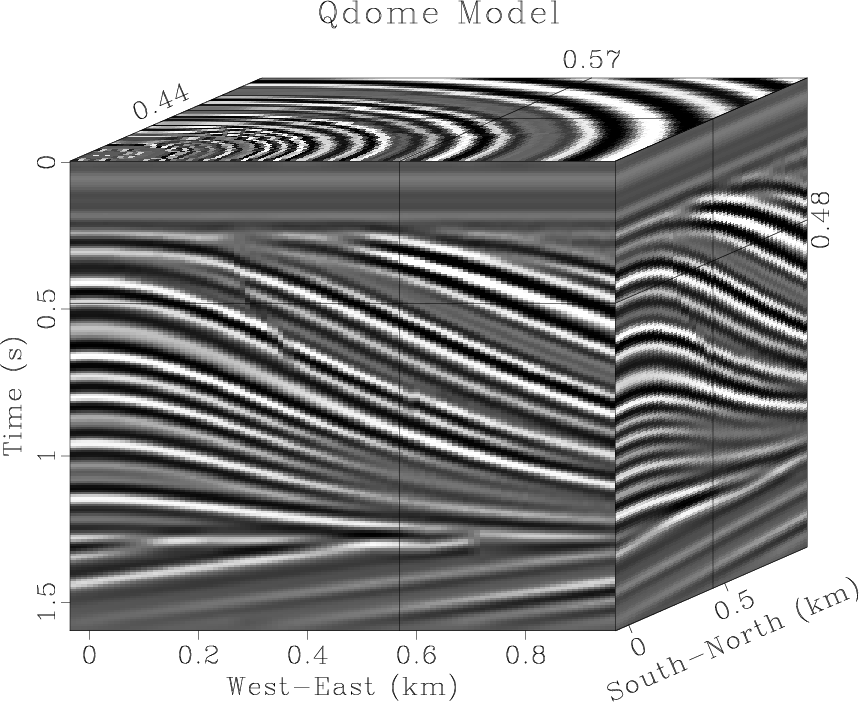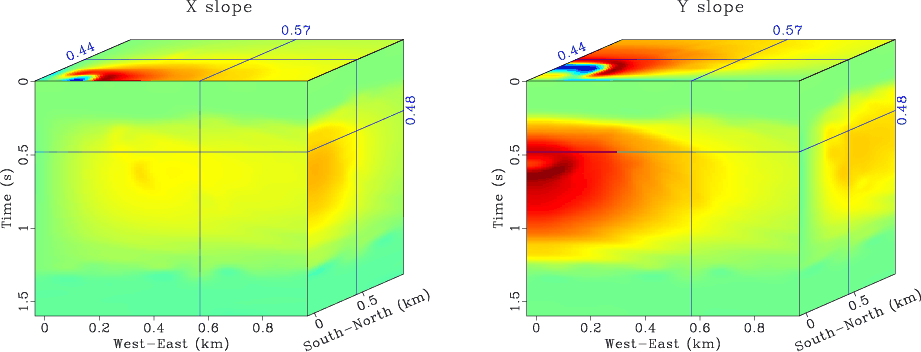## Why does Madagascar have such poor documentation?

June 17, 2012 FAQ No comments

Most likely, the person asking this question does not know where to look for documentation. With 6 schools, 300 blog posts, 80 wiki pages, 500-page manual and 50-page tutorial, there is plenty of information for somebody willing to learn about Madagascar or to understand how it works.

Reproducible papers and books link scientific descriptions of different algorithms with examples of their usage and thus represent the best kind of documentation scientific software may have. On the last count, there were more than 500 computational recipes (SConstruct files) and more than 5,000 reproducible figures. Reproducibility means: we provide reproducible examples of using a particular program but we do not guarantee that the program will work properly with a different choice of parameters or with different input data. With time, the ecosystem evolves. Some programs are getting used more often and, as a result, are getting better debugged and more thoroughly documented.

Madagascar has a low barrier for authors to start contributing their work. When some of the newly contributed programs and examples are not sufficiently well documented, documentation becomes a process of communication between authors and users. Engaging users as active participants in this process will help us make it more efficient.

## IWAVE

June 7, 2012 Systems No comments

A major enhancement of Madagascar is the recent addition of IWAVE, a wave modeling package from Bill Symes and The Rice Inversion Project (TRIP). IWAVE includes includes a variable-density acoustics modeling application, and the initial release of an isotropic elastic modeling code, both with PML boundary conditions, parallelization over loops and tasks, and other features. Its authors are Igor S. Terentyev, Tatyana Vdovina, William W. Symes, Xin Wang, and Dong Sun. IWAVE was used as a benchmarking and QC tool by SEAM, the SEG modeling project.You can see a simple example of using IWAVE with Madagascar in this reproducible paper.

## Program of the month: sfdip

June 2, 2012 Programs No comments

sfdip estimates a local slope (dip) using the plane-wave destruction algorithm.

The dip is measured in time samples. If $\alpha$ is the dip angle, then the output of sfdip corresponds to the dimensionless quantity $p=\tan{\alpha}$.

The following example from jsg/flat/flat shows an input synthetic dataset and an estimated dip fieldWhen applied to 3-D data, sfdip outputs a 4-D file with n4=2 and two dips (inline and crossline). To compute only the inline dip, use n4=0. To compute only the crossline dip, use n4=1. The following example from sep/plane/qint shows an input synthetic 3-D dataset and the two dip components calculated from it.The algorithm consists of a number of non-linear Gauss-Newton iterations (specified by niter=) with a number of linear CG-shaping iterations (specified by liter=) inside each non-linear cycle. The convergence depends on the initial dip values, which can be specified either as constants (p0= and q0=) or as auxiliary input files (idip= and xdip=). If it is necessary to constrain the range of dip values, it can be controlled by specifying minimum or maximum parameters (pmin=, pmax= and qmin=, qmax=). The smoothness of the dip is assured by shaping regularization and controled by the smoothing radii rect1=, rect2=, rect3=.

With default parameters, the dip estimate is accurate only up to 45 degrees. To estimate steeper (aliased) dips, increase the order= parameter. The order of the filter corresponds to the maximum dip. Alternatively, one can use the technique of filter stretching (interlacing), explained by Claerbout; the stretching parameters are nj1= and nj2=. The following examples from sep/pwd/alias show aliased data interpolation using (a) order=12 (b) order=3 nj1=4.For a faster version, with only one non-linear iteration but with fewer options, try sffdip. To estimate two interfering dips, try sftwodip2. To estimate a number of constant dips, try sfdips.